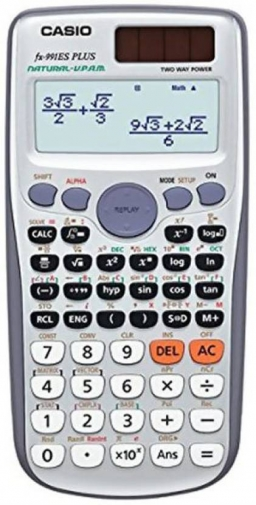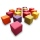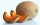# Without 2

Without multipying, tell whether the product 0.644 x 0.25 will be greater than 1 or less than 1? Explane how you know. Then find the product.

p =  0.161

### Step-by-step explanation:Did you find an error or inaccuracy? Feel free to write us. Thank you!## Related math problems and questions:

• How manyHow many integers are greater than 547/3 and less than 931/4?
• RootThe root of the equation (x-19)2 -10 = x2 -11x is (equal or greater or less than zero)? ...
• The length 6The length of 12 pipes is 10 1/2 meters. (1) find the length of one pipe (2) also find the length of 7 pipes
• Sorting ASCWhich group of decimals is in order from least to greatest? A) 1.04, 0.39, 0.8, 2.1, 0.09 B) 2.1, 1.04, 0.39, 0.8, 0.09 C) 0.09, 0.39, 0.8, 1.04, 2.1 D) 0.09, 0.39, 0.8, 2.1, 1.04
• Two-digit numberI am a two-digit number less than 20. When I divide by three, then you get the rest one, and when you divide me by four, you get also rest 1. What number am I?
• Two numbers and its productThe product of two numbers are 2/3. If on of them is 1/10, what is the other?How much and how many times is 72.1 greater than 0.00721?
• Divide 11Divide the product of 4 and 5/8 by 1 1/2. Write your answer as a mixed number.
• Paper boxCalculate whether 11 dm² of paper is sufficient for gluing a box without a lid with bottom dimensions of 2 dm and 15 cm and 12 cm high. Write result as: 0 = No, 1 = Yes
• How manyHow many numbers are less than 222 with a digit sum is 8?
• Find the 24Find the difference between 2/7 and 1/21
• DigitsHow many odd four-digit numbers can we create from digits: 0, 3, 5, 6, 7? (a) the figures may be repeated (b) the digits may not be repeated
• Comparing and sortingArrange in descending order this fractions: 2/7, 7/10 & 1/2
• Two wallsCalculate the surface area of a cube in m2 if you know that the area of its two walls is 72 dm2.
• BeerWhich beer is better to drink: small beer (0.3 L) for 0.67 € or large (0.5 L) for 1.81 €?
• Evaluate 11Multiply the quotient of 6 and 2 by 3, then add 1. Add 1 and 6, then divide by 2 and multiply by 3. Divide 6 by the product of 2 and 3, then add 1.
• Fruits 2An apple costs 7p and a melon costs seven times more. How much will 6 apples and 2 melons cost